## Sunday, January 26, 2014

### TI-84+: BIN2DEC, DEC2BIN, DRAWICON

TI-84+: BIN2DEC, DEC2BIN, DRAWICON

Switching gears for a bit, this blog focuses on the TI-84+:

BIN2DEC: Binary to Decimal: Now allows for any size (up to memory limit).
Input: A list of bits (zeroes and ones). Power of 2 is in descending order from 2^(D-1) to 2^0 where D is the length of the list.

L1: List 1 (2nd, 1; shown as big L with 1 as a subscript)

Example: 11011_2 → 27_10
Input (L1): {1,1,0,1,1}
Output (N): 27

PROGRAM:BIN2DEC
: Input "L1 OF 0 AND 1S:",L1
: dim(L1)→D
: 0→N
: For(K,0,D-1)
: N+2^K*L1(D-K)→N
: End
: Disp "N=DEC"
: Disp N

DEC2BIN: Decimal to Binary
Input: Integer (anything after the decimal point is ignored)
Output: List of binary bits. Power of 2 is in descending order from 2^(D-1) to 2^0 where D is the length of the list.

Example: 5427_10 → 1010100110011_2
Input (N): 5427
Output (L1): {1,0,1,0,1,0,0,1,1,0,0,1,1}

PROGRAM:DEC2BIN
: Input "N:", N
: If N<0
: Then
: Disp "INVALID"
: Stop
: End
: iPart(N)→M
: iPart(ln(M)/ln(2))+1→D
: DelVar L1
: D→dim(L1)
: For(K,0,D-1)
: If 2^(D-1-K)≤M
: Then
: 1→L1(K+1)
: M-2^(D-1-K)→M
: End
: End
: Disp "L1=BIN"
: Pause L1

DRAWICON: Draws a 5 × 5 pixelated pictures using an integer from 1 to 33,554,431. The number is converted into its binary bits. The upper left hand corner represents 2^24. The exponent decreases going right then down each row, with 2^0 representing the bottom right hand corner.

Matrix: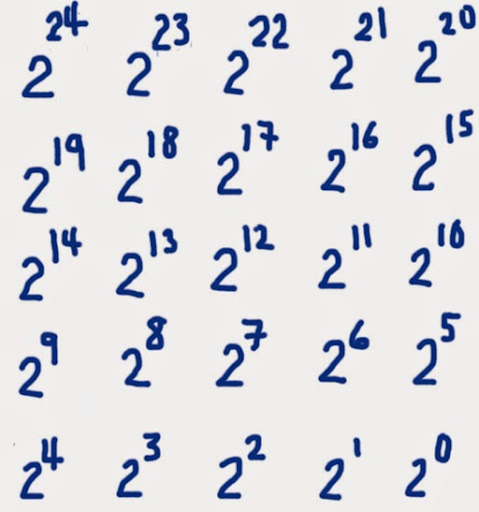PROGRAM:DRAWICON
: 24→N
: Input "NUMBER < 2^25:", M
: If M>2^25-1
: Then
: Disp "EXCESS"
: Stop
: End
: iPart(M)→M
: ClrHome
: For(R,1,5)
: For(C,1,5)
: If 2^N≤M
: Then
: Output(R,C,"X")
: M-2^N→M
: End
: N-1→N
: End
: End
: Pause
: ClrHome

Below are a few examples: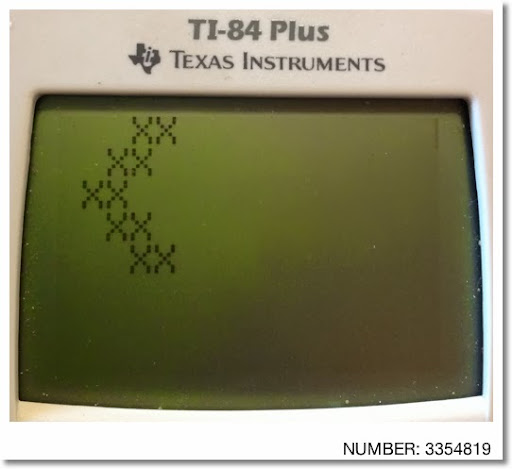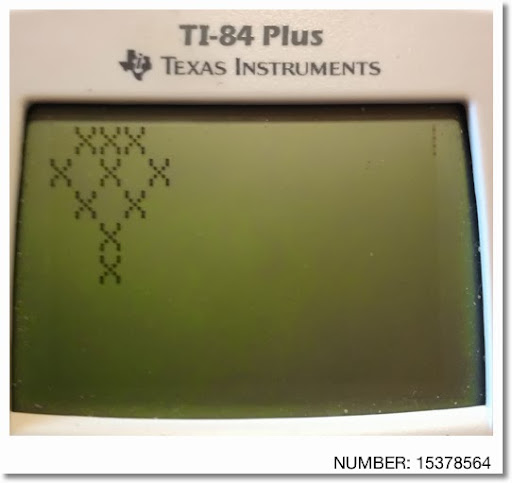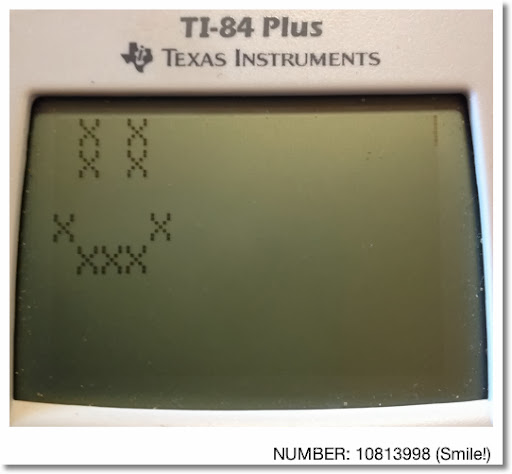Enjoy, and don't forget to make each day count! Until next time,

Eddie

This blog is property of Edward Shore. 2014

## Tuesday, January 21, 2014

### HP Prime and HP 32SII: Day Number of the Year

HP Prime and HP 32S: Day Number of the Year

This formula calculates the day number of any date (given month and day). A 365 day year is used. If you are operating with a leap year with dates after February 28, add 1.

Hence in non-leap years, January 1 is Day 1, February 1 is Day 32, etc..., December 1 is 335, and December 31 is 365.

Derivation

Formula Used: Gregorian Calendar

Let: Y = year, D = day, and M = month. Then:

N = IP(365T) + IP(T/4) - IP(T/100) + IP(T/400) + D + IP((153X + 8)/5)

where IP represents the integer portion of number and:

if M≤2, T=Y-1, X=M+12,
otherwise T=Y and X=M.

Source: Dr. Math ( http://mathforum.org/library/drmath/view/66857.html )

To find the day number, use the above formula to get the "Gregorian" day number (better term?) for the date in question and subtract the "Gregorian" day number for January 1. This gives the number of days between January 1 and said date. Since the days between dates does not include January 1, we need to include January 1 by adding 1.

For derivation purposes, let's calculate the days between January 1, 1 (AD) and target month and date of year 1. Add 1 to include January 1. I arbitrary chose 1 as the year for ease of calculation and the "year 1" would have been a 365 day year.

Day Number from January 1 to February 28 (29 for leap years)

Let M = month number (1 for January, 2 for February), D = date number

Day Number: (N_(M,D) - N_(1,1)) + 1

Since M≤2, T=1-1=0 and X=M+12

(IP(365*0) + IP(0/4) - IP(0/100) + IP(0/400) + D + IP((153(M+12)+8)/5)
- (IP(365*0) + IP(0/4) - IP(0/100) + IP(0/400) + 1 + IP((153(1+12)+8)/5)) + 1

= (D + IP((153M+1836+8)/5) - (1 + IP(1997/5))) + 1

= (D + IP(30.6M + 368.8) - (1 + 399)) + 1

= D + IP(30.6M + 368.8) - 399

Day Number for March 1 to December 31

Since M>2, T=Y and X=M. Then, again assuming Y=1:

(IP(365*1) + IP(1/4) - IP(1/100) + IP(1/400) + D + IP((153M+8)/5))
- (IP(365*0) + IP(0/4) - IP(0/100) + IP(0/400) + 1 + IP((153(1+12)+8)/5)) + 1

= (365 + D + IP(30.6M + 1.6)) - (1 + IP(1997/5)) + 1

= (365 + D + IP(30.6M + 1.6) - 399

= D + IP(30.6M + 1.6) - 34

Summary:

Day Number, 365 Day Year, M≤2:
D + IP(30.6M + 1.6 + 367.2) - (34 + 365) = D + IP(30.6M + 368.8) - 399

M>2:
D + IP(30.6M + 1.6) - 34

HP Prime Program DAYNO

EXPORT DAYNO(M,D)
BEGIN
IF M≤2 THEN
RETURN IP(30.6*M+368.8)+D-399;
ELSE
RETURN IP(30.6*M+1.6)+D-34;
END;
END;

Here it is in RPN format. I broke up some of the larger numbers into an addition of two smaller ones and used flags to keep the program within one label.

HP 32SII

N01 LBL N
N02 CF 0 \\clear flag 0 (any user flag will do)
N03 INPUT M
N04 2
N05 x≥y?
N06 SF 0 \\set flag 0
N07 R-down \\bring M to the X stack
N08 30.6
N09 ×
N10 1.6
N11 +
N12 FS? 0
N13 367.2
N14 FS? 0
N15 +
N16 IP
N17 INPUT D
N18 +
N19 34
N20 -
N21 FS? 0
N22 365
N23 FS? 0
N24 -
N25 CF 0
N26 RTN

Eddie

This blog is property of Edward Shore. 2014

## Friday, January 17, 2014

### HP Prime and TI-84+: Chinese Remainder Theorem - Solving Three Congruence Equations

Chinese Remainder Theorem - Sun Tzu (3rd to 5th century AD, China)

This program solves for X for the set of three linear congruence equations:
X = A mod B
X = C mod D
X = E mod F

B, D, and F are pairwise co-prime. B and D have a GCD of 1, B and F have a GCD of 1, and D and F have a GCD of 1.

A general solution takes the form
X = x1 * D * F * A + x2 * B * F * C + x3 * B * D * E

where (one method of solution)
D * F * x1 = 1 mod B
B * F * x2 = 1 mod D
B * D * x3 = 1 mod F

The program returns the solution X ± n*(B*D*F), X is the smallest positive integer and n is an integer.

Sources:
Chinese Remainder Theorem - General Information:
http://en.wikipedia.org/wiki/Chinese_remainder_theorem

Chinese Remainder Theorem - One method of solution:
http://youtu.be/3PkxN_r9up8

Solving a Linear Congruence Equation:
http://youtu.be/U9Eo6Bsvm4M

Examples:

X = 3 mod 8
X = 1 mod 9
X = 4 mod 11
X = 235 ± 792*n
{...-557, 235, 1027, 1819...}

X = 4 mod 5
X = 1 mod 7
X = 11 mod 16
X = 379 ± 560*n
{... -181, 379, 939, 1499...}

HP Prime Program CHINESE3
Output: [ X, B*D*F ]

// Declare subroutine
SUB1( );

// Main routine
EXPORT CHINESE3( )
BEGIN
LOCAL A,B,C,D,E,F,I,T:=0;

INPUT({A,B,C,D,E,F}, "X=A mod B=C mod D
=E mod F");

T := SUB1(D*F, B) * D * F * A + SUB1(B*F, D) * B * F * C
+ SUB1(B*D, F) * B * D * E;
T := T MOD (B*D*F);
RETURN [T, B*D*F];
END;

SUB1(X,Y)
BEGIN

FOR I FROM 1 TO X-1 DO
IF FP((X-1)/Y)==0 THEN
RETURN I;
END;
END;

RETURN "NO SOLUTION";

END;

TI-84+:
Main Routine: CHINESE3
Subroutine: CHIN3SUB
Output:
X
+/- N*
B*D*F

PROGRAM:CHINESE3
: ClrHome
: Disp "X=A MOD B"
: Disp "X=C MOD D"
: Disp "X=E MOD F"
: Prompt A,B,C,D,E,F
: 0 → T
: D * F → X : B → Y :prgmCHIN3SUB
: I * D * F * A → T
: B * F → X : D → Y : prgmCHIN3SUB
: T + I * B * F * C → T
: B * D → X : F → Y : prgmCHIN3SUB
: T +I * B * D * E → T
: remainder(T, B * D * F) → T
: Disp T
: Disp "+/- N*"
: Disp B * D * F

PROGRAM:CHIN3SUB (Subroutine)
: For(I, 1, X-1)
: fPart((X*I-1)/Y) → Z
: If Z=0
: Then
: Return
: End
: End
: Disp "NO SOLUTION"
: Stop

## Wednesday, January 15, 2014

### Video: The Pi Episode by Danica McKellar and Nerdist

Major thanks to Dr. Cliff Pickover for posting the link to this awesome video on my Twitter feed.  On January 9, 2014, Danica McKellar premiered her YouTube show "Math Bites".  The first show is all about pi (π).  OK, they talk about pie too, but the main focus is one the famous number.

From memory, I know π = 3.14159265353897923846...  (I think that's right).  Anyway, click on the link and enjoy!

And not only my favorite number is π, my favorite pie is apple, especially if it has cinnamon with a crust on top.

If you are on Twitter (who isn't these days?):

Follow Danica McKellar, author of "Math Doesn't Suck", "Hot X: Algebra Exposed", "Kiss My Math: Showing Pre-Algebra Who's Boss" and "Girls Get Curves: Geometry Takes"  - @danicamckellar

Follow Chris Hardwick, head of Nerdist and supremely funny guy.  He also has his Nerdist podcasts.  Too bad I don't have a burrito around to enjoy while I'm typing this. Oh, he hosts Talking Dead (The show which discusses "The Walking Dead") and @midnight  - @nerdist

Follow Dr. Cliff Pickover, scientist, mathematician, and writer.  His books include "The Math Book" and "The Physics Book" - @pickover

And I have a Twitter account too, mine is @edward_shore.

Eddie

Next post will be post the 300th of this blog!  Again, I thank you for your support - much appreciated.

### HP Prime and HP 32S: Solving a Single Linear Congruence Equation

Happy Mid-January! Enjoying the first full moon of 2014?

Solving a Single Linear Congruence Equation

The program solves for x in the equation:

A * x = B mod N

Examples:

4 * x = 6 mod 7
A = 4, B = 6, N = 7
Solution: 5

5 * x = 3 mod 17
A = 5, B = 3, N = 17
Solution: 4

11 * x = 3 mod 16
A = 11, B = 3, N = 16
Solution: 9

HP Prime Program: CONG

EXPORT CONG( )
BEGIN
LOCAL A,B,N,I;
// 2014-01-15 EWS

INPUT({A,B,N}, "Ax = B mod N",
{"A","B","N"}, { }, {0, 0, 0} );

// safe guard if the user does not enter integers (optional line)
A:=IP(A); B:=IP(B); N:=IP(N);

// Algorithm
FOR I FROM 1 TO N-1 DO

IF FP((A*I-B)/N) == 0 THEN
MSGBOX("x ="+STRING(I));
RETURN I;
KILL;
END;

END;
RETURN "No Solution";
END;

HP 32S Program: CONG

Main Routine: Label C
Subroutines: D, E
No pre-storing any variables required. If there is no solution, an error is produced.
To Run: XEQ C

Program:
C01 LBL C
C02 INPUT A
C03 INPUT B
C04 INPUT N
C05 1
C06 STO I

D01 LBL D
D02 RCL I
D03 RCL × A
D04 RCL - B
D05 RCL ÷ N
D06 FP
D07 x ≠ 0?
D08 GTO E
D09 RCL I
D010 RTN

E01 LBL E
E02 1
E03 STO + I
E04 RCL I
E05 RCL N
E06 x > y?
E07 GTO D
E08 0
E09 1/x
E10 RTN

I am working on a program for the Chinese Remainder Theorem for 2 or 3 linear congruence equations, to be posted soon (hopefully).

Have a great day!

Eddie

This blog is property of Edward Shore. 2014

## Saturday, January 11, 2014

### HP Prime: Waterfall Animation

HP Prime: AMNI10
Waterfall Animation

Background boxes with falling water. There is random mist coming from the water.
Colors:
Blue: #FFh, RGB(0,0,255)
White: #FFFFFFh, RGB(255,255,255)
Brown: #964B00h, RGB(150,75,0)
Grass Green: #DA00h, RGB(0,218,0)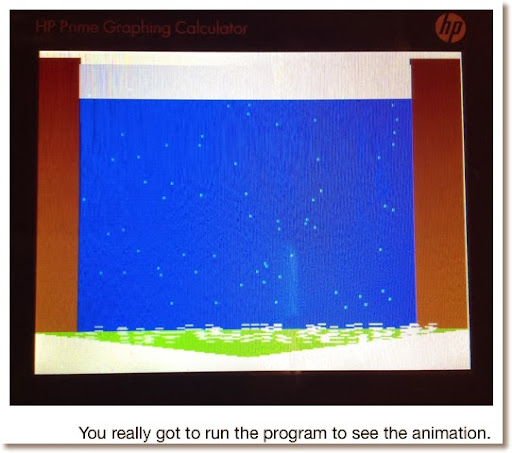EXPORT AMNI10()
BEGIN
// 2014-01-10 Waterfall EWS
LOCAL K,J,I,A:=0;
RECT();

WHILE GETKEY==-1 DO
A:=A+1;

// Static
// Walls
RECT_P(0,5,30,210,#964B00h);
RECT_P(280,5,318,210,#964B00h);
// Water
RECT_P(30,10,280,210,#FFh);
// Grass
TRIANGLE_P(0,210,155,230,318,210,
RGB(0,218,0));

// Animated
// Mist
FOR I FROM 1 TO RANDINT(50,75) DO
TEXTOUT_P(".",RANDINT(30,270),
RANDINT(10,200),3,#FFFFh); END;
// Foam
FOR I FROM 1 TO RANDINT(100,125) DO
TEXTOUT_P("=",RANDINT(25,290),
RANDINT(200,210),3,#FFFFFFh); END;
// Fall
RECT_P(30,10+25*(A+1),280,10+25*A,
#FFFFFFh);
WAIT(0.1);
IF A==8 THEN
A:=0; END;

END;
END;
I realize this is more a picture one would see when running an Atari 2600 game.  I guess the point is how to layer graphics so that the static elements are drawn first, then the animated elements.

Eddie

This blog is property of Edward Shore. 2014

### HP Prime: Area of a Fan-Shaped Field (Baseball Field)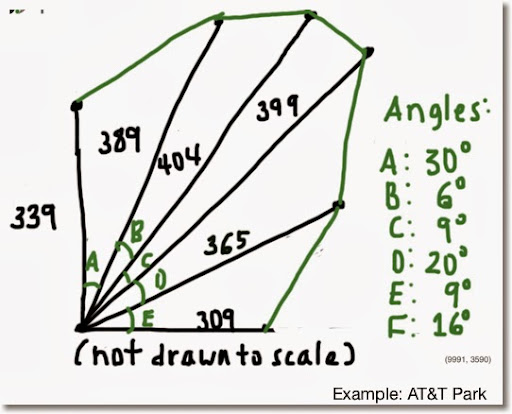Given the lines of various length from a single vertex and the angles between the lines, the area of the field can be calculated.

FANAREA(list of lengths, list of angles in degrees)

The result is a list of two elements.
First element: area of the field
Second element: list: length of the connecting lines

Example:
AT&T Park (see the above figure)
Dimensions from Clem's Blog:
http://www.andrewclem.com/Baseball/ATTPark.html

Angles measured with a protractor. So, realistically, my estimate of area is rough.

L1:={339,382,404,399,421,365,309}
L2:={30,6,9,20,9,16}
FANAREA(L1,L2) returns
{109337.870804, {191.1175010192, 46.6352856001, 63.1995292625,
144.030366611, 83.1849883392, 108.96879942}}

Area of the field: approximately 109,337.871

Note: CHAR(10) gives a line break in a string.

PROGRAM:

// Declare Subs
SUB1();
SUB2();

EXPORT FANAREA(L1,L2);
BEGIN
// 2014-01-11 EWS
// Area of a field
// lines from vertex w/angles
LOCAL I,T:=0,L3:=L2;
// Degree
HAngle:=1;

// Validate
IF SIZE(L1)≠SIZE(L2)+1 THEN
MSGBOX("Error: Wrong"+CHAR(10)
+"Size"+CHAR(10)
+"L2≠L1+1");
KILL; END;

// Calculation
FOR I FROM 1 TO SIZE(L2) DO
L3(I):=SUB1(L1(I),L1(I+1),L2(I));
T:=T+SUB2(L1(I),L1(I+1),L3(I));
END;

MSGBOX("Area: "+T);
RETURN {T, L3};
END;

// Law of Cosines
SUB1(A,B,C)
BEGIN
RETURN √(A^2+B^2-2*A*B*COS(C));
END;

//Heron's Formula
SUB2(A,B,C)
BEGIN
LOCAL S:=(A+B+C)/2;
RETURN √(S*(S-A)*(S-B)*(S-C));
END;

## Friday, January 10, 2014

### Geometry: Area of a Cone-Shaped Field

Honestly the name of the object presented on this blog entry is made up names because I don't think there is an official geometrical name. This was inspired by trying to find areas of baseball fields. Hopefully this provides inspiration and maybe insight.

Area of a Cone-Shaped Field

Description: A triangle with line segments splitting away from each other at an 90° angle. After a certain distance, where the triangle stops, and half-ellipse is attached at the end.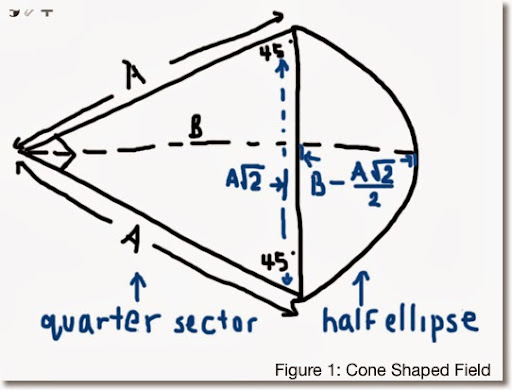Measurements given:
A = length of a vector
B = length of a segment from the vertex to the outer point of the half ellipse. This line divides the shape in symmetric halves on each side.

The area of the cone-shaped field can be found in two parts:
(I) Area of the triangle
(II) Area of the half-ellipse
Total Area = (I) + (II)

Part (I):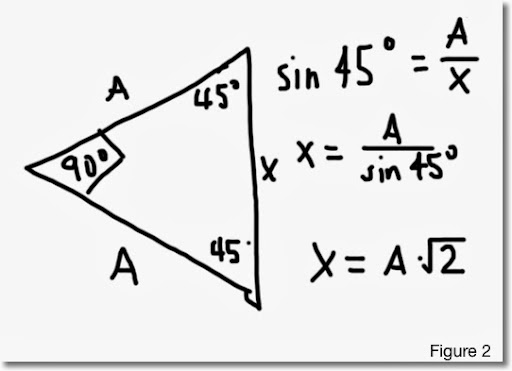Let X be the length of the line connecting the end point of the out reaching lines. (See Figure 2)

Then from trigonometry, sin 45° = A/X which implies that X = A*√2. This will come in handy for part (II).

Taking advantage of the fact that the triangle is a right triangle, we can use the basic area formula 1/2 * base * height and the area of (I) is:

1/2 * A^2.

(II) Finding the area of the half ellipse. Let's start with the general formula
π * length of the semi-major axis * length of the semi-minor axis.

Since we are working with half of the ellipse, the general formula becomes
1/2 * π * length of the semi-major axis * length of the semi-minor axis.

I am not worried whether which of the axis is the major and which one is the minor. I am going for a general formula.

From Figure 2 above, X = A*√2. This is one of the axis. Half of this length is 1/2*A*√2.

Take a look at Figure 3 below to see how we will obtain the length of the other axis: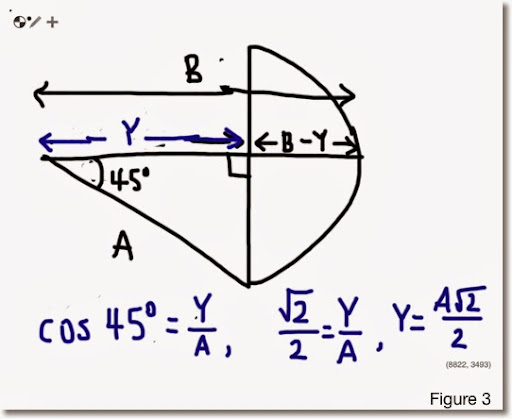Break the length B into two parts. Let Y be the length of the line segment from the vertex to the end of the triangle. For finding the area of the half ellipse, we want the quantity B - Y, which represents the length of one of the semi-axis.

The line bisects the 90° angle into two 45° angles. Using trigonometry, Y = A*√2/2.

Hence, our semi-axis length is B - Y = B - A*√A.

Then the area of the half-ellipse is:

1/2 * π * (A*√2/2) * (B - A*√2/2)
= π/4 * (A*B*√2 - A^2)

Having the two parts completed, the total area of the cone-shaped field is:

(I) + (II) = A^2/2 + π/4 * (A * B * √2 - A^2)

The take away point is that if you have an unusual shape and if you can recognize at least parts of familiar shapes, you can come up with a fairly closed formula for calculating the area for that shape.

Until then, have a great day and get creative!

Eddie

This blog is property of Edward Shore. 2014

## Tuesday, January 7, 2014

### Probability: Odds of Winning at Slot Machines

The Odds of Hitting it Big

The number of possible combinations is fairly easy to calculate. You multiply the number symbols each slot has together. For example for a three slot machine with six symbols a piece, the number possible combinations is 6 × 6 × 6 = 216. Although I have never played on one (knowingly), you could have a slot machine were each slot itself has a different amount of symbols.

Calculating the probability of winning on a slot machine is fairly simple. The odds of getting a certain combination is:

(number of winning combinations)/(number of possible combinations)

Different winning combinations offer different playoffs. The harder the combination, the bigger the reward. In gambling, the term payout percent is used when describing how "lucrative" the slot machine is. In general it is calculated like this:

Σ(winning combination_k * payoff_k)/(number of possible combinations)

Note that is different from the total of number of winning combinations over the number of possible combinations ratio. The payoff acts as a weight for each winning combination. (It is like weighted average, but not quite.)

Let us look at analyzing a pair of slot machines. For this blog entry, we will assume that the slot machine has one line and takes only one coin per roll.

Analyzing a Simple Slot Machine

The first machine has three slots. On each slot there is an apple, orange, lemon, banana, melon, and a Joker. The payouts are as follows:

(I) Three Jokers (Joker | Joker | Joker) pays 30 coins
(II) Any Three of the same fruit (example: Apple | Apple | Apple) pays 10 coins
(III) Any Two Jokers pays 4 coins
(IV) Any One Joker pays 1 coin (break even)

Number of Possible Combinations: 6 × 6 × 6 = 216

Number of Winning Combinations (see above):

(I): There is only one configuration for this: three Jokers.

(II): Three of a kind that isn't a Joker. One the first slot, you can get any of the five fruit (apple, orange, lemon, banana, melon). Once that fruit is selected, that fruit has to match on the next two slots. So, there are 5 such combinations: three apples, three oranges, three lemons, three bananas, and three lemons.

(III): Jokers can appear any two slots: slots 1 and 2, slots 1 and 3, and slots 2 and 3. The other slot must contain fruit. Hence the total number of winning combinations possible is 1 × 1 × 5 + 1 × 5 × 1 + 5 × 1 × 1 = 15.

(IV): Jokers can appear in any slot, the other two slots must have fruit. In this instance, it does not matter if the other two slots match. The number of combinations is 1 × 5 × 5 + 5 × 1 × 5 + 5 × 5 × 1 = 75.

There are a total of 1 + 5 + 15 + 75 = 96 winning combinations. The table below shows the calculation of slot's payout.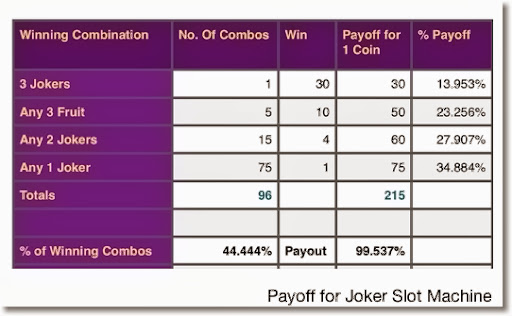Calculating the payoff percentage:

(1 × 30 + 5 × 50 + 15 × 4 + 75 × 1)/(6 × 6 × 6)
= 215/216
≈ 0.99537

The payoff percentage is 99.537%. That is pretty good in terms of slot machines. It could be a little misleading given that the harder combinations are given more weight.

Had everything paid equally, only 96/216, or about 44.444% of the combinations would win. I doubt no one would play a slot machine with less than a 50% chance of spinning a winning if there was no added incentive, such as a richer payoff.

A More Complex Slot Machine

We are still at one line, three slots. Here is the slot distribution: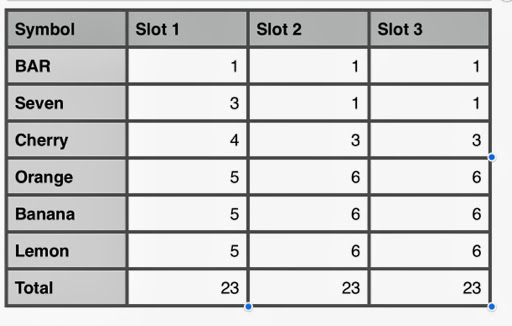There are 23 symbols per slot. So the number of possible combinations is 23 × 23 × 23 = 12,167.

Also note that the non-equal distribution of symbols on each slot. With the lack of BARs, cherries, and Sevens (7), it is obvious that getting winning combinations involving these three symbols offer higher playoffs.

The winning combinations, with payoffs are: (See the table above for the distribution of symbols)

(I) 3 BARs. Wins 60 coins. Number of winning combinations: 1

(II) 3 Sevens. Wins 40 coins. Number of winning combinations: 3 × 1 × 1 = 3

(III) 3 Cherries. Wins 20 coins.
Number of winning combinations: 4 × 3 × 3 = 36

(IV) 3 of any Fruit (3 Oranges, 3 Bananas, 3 Lemons)
Wins 10 coins.
Number of winning combinations:
For Oranges: 5 × 6 × 6 = 180
For Bananas: 5 × 6 × 6 = 180
For Lemons: 5 × 6 × 6 = 180
Total: 540

(V) Cherries in Any Two Slots:
Wins 4 coins.
Number of winning combinations:
Cherry, Cherry, Other: 4 × 3 × (23-3) = 240
Cherry, Other, Cherry: 4 × (23-3) × 3 = 240
Other, Cherry, Cherry: (23-4) × 3 × 3 = 171
Total: 651

(VI) Cherry in any one slot:
Wins 1 coin.
First Slot: 4 × 20 × 20 = 1600
Second Slot: 19 × 3 × 20 = 1120
Third Slot: 19 × 20 × 3 = 1120
Total: 3880

The following table calculates the payoff percentage for this machine: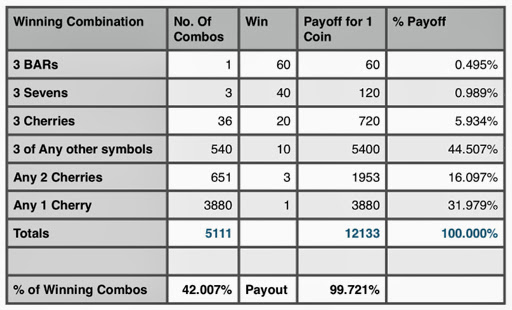This second table has a payoff percentage of 99.721%. If everything paid 1 coin equally, it would drop to just over 42%.

For more complex slot machines (more slots and multiple lines), the use of a spreadsheet or other computer program will come to the aid of calculating payoff since the number of calculations grow with complexity.

Gamble responsibly!

Eddie

Source:
Slot Machine Math - Gambler's Bookcase
http://www.gamblersbookcase.com/Slots-Math.htm

This blog is property of Edward Shore. 2014

## Sunday, January 5, 2014

### Playing Cards: Odds That The Next Card is Higher or Lower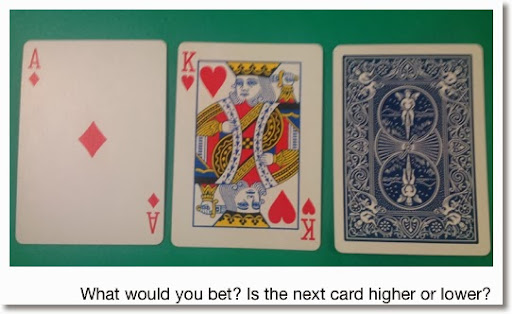In the classic game "Card Sharks", contestants had to guess and wager whether the proceeding card is higher or lower. The card game is popular to watch on TV and to play on yourself. All it takes a deck of cards to play.

This blog entry deals with the odds of whether the next card is higher or lower. Several assumptions: the odds are calculated with a standard 52 card deck without Jokers. The tables deal with the first cards drawn from the top of the deck.

Table 1: One card is drawn from the top of the deck.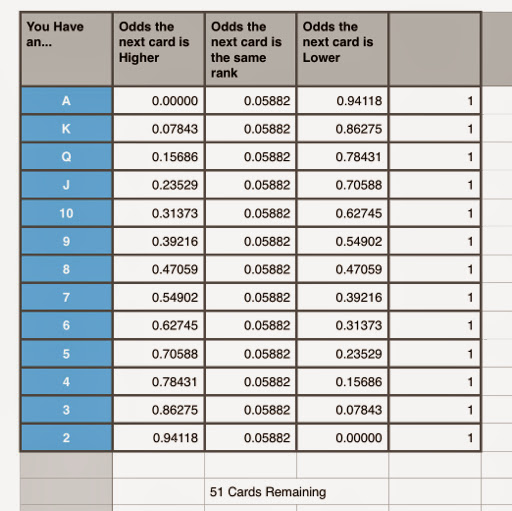Table 2: One card is drawn. A second drawn which is lower than the first card. Examples: Ace then King, Ten then Six.

The second card is the current card.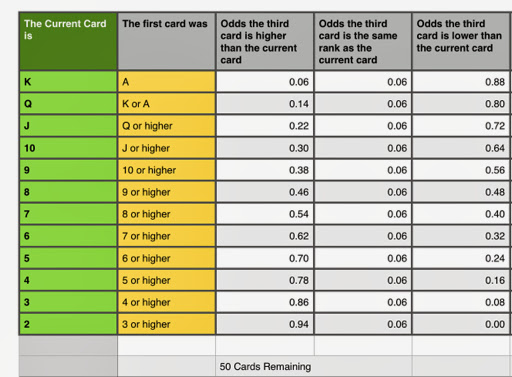Table 3: One card is drawn. A second drawn which is higher than the first card. Examples: King then Ace, Six then Ten.

The second card is the current card.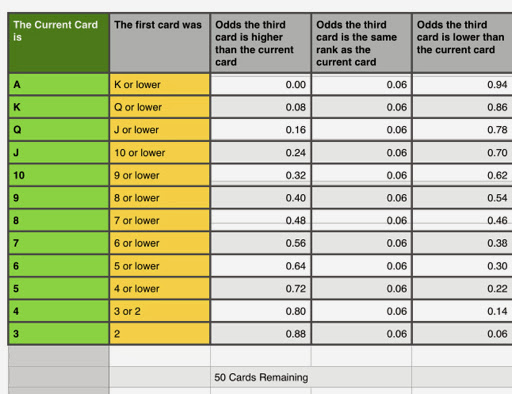Table 4: A pair of cards are drawn. Example: A pair of Eights.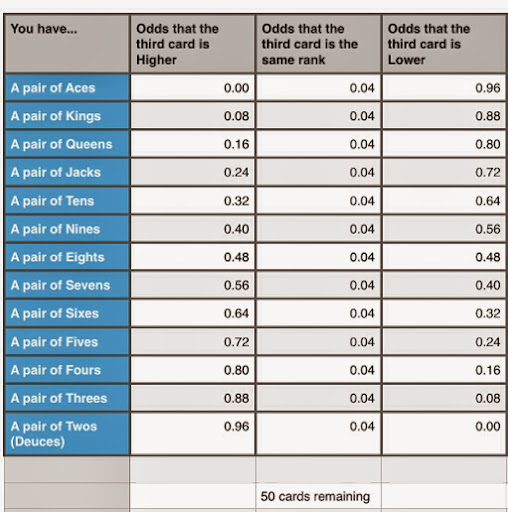General strategies of Card Sharks consisting of:
* Betting a lot on lower when you have an Ace or King
* Betting a lot on higher when you have a 2 or 3
* Betting minimum on a 7, 8, or 9 (middle ranks)

Analyzing a Hand

In general, the odds of whether cards are higher or lower as the number of cards decrease. Let's see how the odds change during a 8 card hand.

** Odds are rounded to 3 digits in this analysis.

Here is an Example Hand:

1st Card: King
Odds the next card is higher: 0.078
Odds the next card is another King: 0.059
Odds the next card is lower: 0.863

2nd Card: 10
Odds the next card is higher: 0.300
Odds the next card is another 10: 0.060
Odds the next card is lower: 0.640

3rd Card: 2
Odds the next card is higher: 0.939
Odds the next card is another 2: 0.061
Odds the next card is lower: 0.000

4th Card: 6
Odds the next card is higher: 0.624
Odds the next card is another 6: 0.063
Odds the next card is lower: 0.313

5th Card: 4
Odds the next card is higher: 0.787
Odds the next card is another 4: 0.064
Odds the next card is lower: 0.149

6th Card: 4
Odds the next card is higher: 0.805
Odds the next card is another 4: 0.043
Odds the next card is lower: 0.152

7th Card: King
Odds the next card is higher: 0.089
Odds the next card is another King: 0.044
Odds the next card is lower: 0.867

8th Card: 5
(The game ends).

Shuffle up and deal!

Eddie

This blog is property of Edward Shore. 2014

### HP 32S II Statistical Formulas

HP 32S II Statistical Formulas Statistics Formulas The 32S II can store formulas for evaluations, including formulas involving stat variable...